## How to find the the "constant is zero" in SAS system ?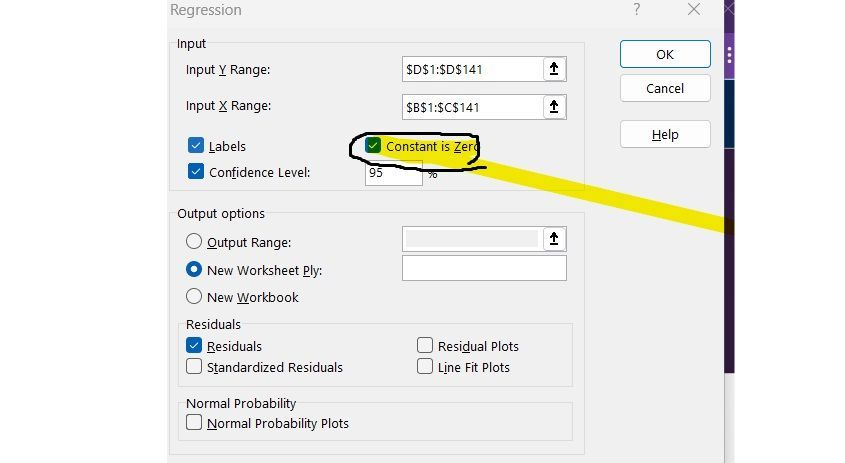Hello I 'am new ,I need to help !!How to find the the "constant is zero" in SAS system ?

12 REPLIES 12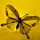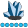whymath
Lapis Lazuli | Level 10

## Re: How to find the the "constant is zero" in SAS system ?

There is a "noint" option when you are using "model" statement in SAS.

## Re: How to find the the "constant is zero" in SAS system ?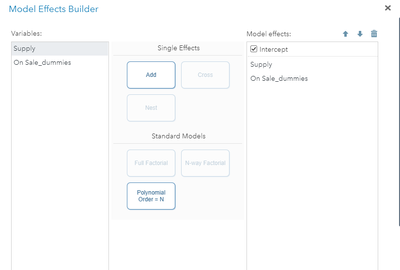I can not find that..

## Re: How to find the the "constant is zero" in SAS system ?

Thank you!! I still can not find that...😭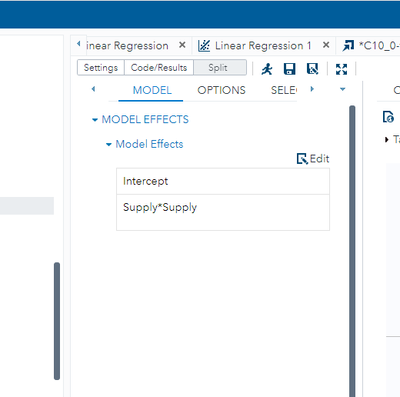## Re: How to find the the "constant is zero" in SAS system ?

Just Un-check the Intercept on the Edit Model screen

## Re: How to find the the "constant is zero" in SAS system ?

Thank you very much!!

## Re: How to find the the "constant is zero" in SAS system ?

I point out that there are times when using no intercept/"constant is zero" is a very poor choice. In fact, it is rare when it is the right choice. So unless you have a good reason for using no intercept/"constant is zero", don't do it.

--
Paige Miller

## Re: How to find the the "constant is zero" in SAS system ?

Because my first mode was bad so I want to remove the intercept.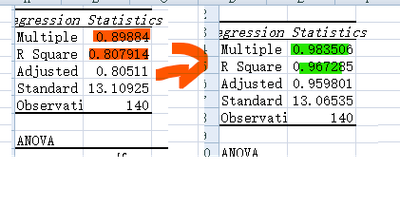## Re: How to find the the "constant is zero" in SAS system ?

0.8 is a decent model, 0.98 is a model is likely overfit.

## Re: How to find the the "constant is zero" in SAS system ?

When I working in the SAS system the answer show looks different,I saw the coefficient intercept p value is 0.78(over 0.05) which suggests that the intercept variable is not statistically significant.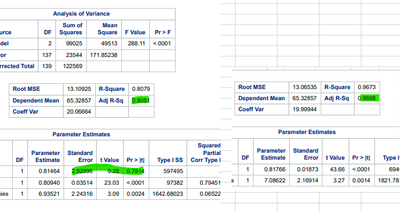## Re: How to find the the "constant is zero" in SAS system ?

@Olivia0223 wrote:

When I working in the SAS system the answer show looks different,I saw the coefficient intercept p value is 0.78(over 0.05) which suggests that the intercept variable is not statistically significant.

Intercept not significant is not really a valid reason to remove it from the model. You are forcing the model through the point x=0 y=0 which may not be a good thing to do in most cases — this is not about statistical significance. You cause the other parameter estimates to be biased; and the residuals will not add to zero. https://stats.stackexchange.com/a/7950

Lastly, just do a plot of the fit between the two different regressions. Here's made up data, we add a certain amount of soap to a dish of water, agitate the water, and then measure the height of the suds formed.

``````data suds;
input soap suds;
cards;
0.6 1.1
0.7 1.2
0.8 1.33
0.9 1.39
1 1.47
1.2 1.6
;
title 'Model with Intercept';
proc reg data=suds;
ods select fitplot;
model suds=soap;
run; quit;
title 'Model without Intercept';
proc reg data=suds;
ods select fitplot;
model suds=soap/noint;
run; quit;``````

Here are the plots, by looking at them, which do you think fits better?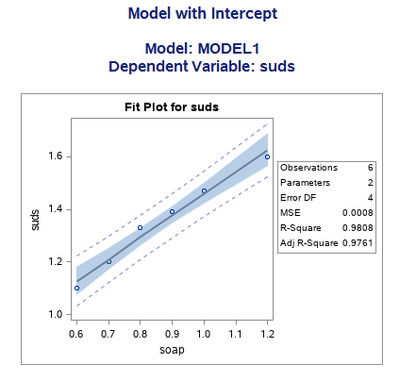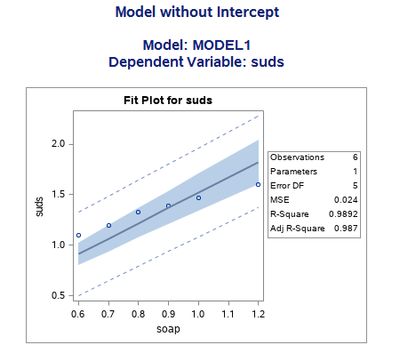--
Paige Miller

## Re: How to find the the "constant is zero" in SAS system ?

It has been a long time since I did the math, but I believe that R-squared without an intercept has to be interpreted differently than with an intercept, and so the two R-squared values are not directly comparable.

Adding: here is a discussion, which includes the math, explaining why you shouldn't compare R-squared with intercept to R-squared without intercept. https://stats.stackexchange.com/questions/26176/removal-of-statistically-significant-intercept-term-...

--
Paige Miller

## Re: How to find the the "constant is zero" in SAS system ?

thank you for your explaining, let me think about how to fix my model.😂

Discussion stats
• 12 replies
• 402 views
• 11 likes
• 5 in conversation